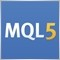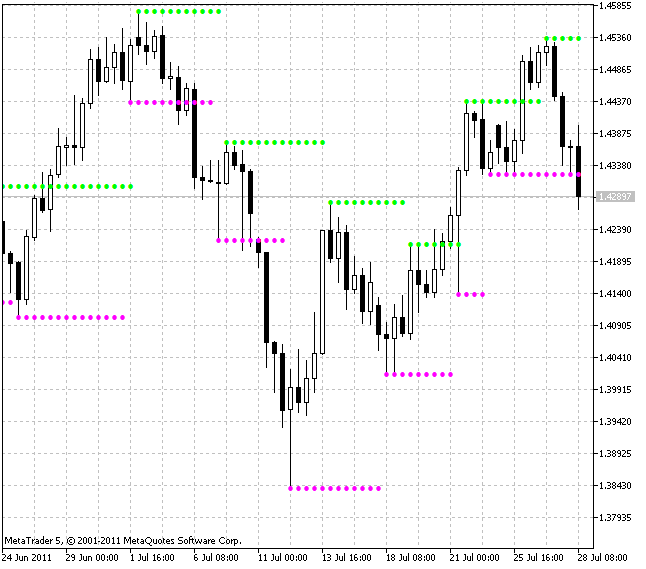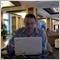• Get access

# Indicators: Support and Resistance153140

The "Support and Resistance" indicators shows the support and resistance levels using the Fractals indicator.

The calculation of support levels is based on the "Fractals down", the calculation of the resistance levels is based on the "Fractals up" of the Fractals indicator by Bill Williams.Author: Nikolay KositsinModerator
11477432

Since the last update of MT5 this indicator is broken. It just prints dots on the high and low of every candle.

What's wrong?39065

yohmm:

Since the last update of MT5 this indicator is broken. It just prints dots on the high and low of every candle.

What's wrong?

This indicator is working normally. Build 1016.32

Yes, I have Build 1016. I downloaded the indicator again and recompiled it with 0 error(s) and 0 warning(s). Still doesn't work, just shows the dots on the high and low.

But it's ok, I am using Fibo retracement or drawing s/r levels on my own now instead.976

yohmm:

Yes, I have Build 1016. I downloaded the indicator again and recompiled it with 0 error(s) and 0 warning(s). Still doesn't work, just shows the dots on the high and low.

But it's ok, I am using Fibo retracement or drawing s/r levels on my own now instead.32

belido:

Sure, here it is:

EURUSD, M5, 2014.11.28

MetaQuotes Software Corp., MetaTrader 5, Demo

temp_file_screenshot_27398.png5

i have the same problem! a reinstall of metatrader does not help allso..:-(
Files:
sup.png 36 kb6

Try this

//+------------------------------------------------------------------+
//|                                       Support and Resistance.mq5 |
//|                                       Update Dec 2014            |
//+------------------------------------------------------------------+
//---- version
#property version   "1.01"
//---- indicator in the chart window
#property indicator_chart_window
//---- 2 indicator buffers are used
#property indicator_buffers 2
//---- 2 graphic plots are used
#property indicator_plots   2
//+----------------------------------------------+
//|  Bearish indicator options                   |
//+----------------------------------------------+
//---- drawing type as arrow
#property indicator_type1   DRAW_ARROW
//---- Magenta color
#property indicator_color1  Magenta
//---- Line width
#property indicator_width1  1
//---- Support label
#property indicator_label1  "Support"
//+----------------------------------------------+
//|  Bullish indicator options                   |
//+----------------------------------------------+
//---- drawing type as arrow
#property indicator_type2   DRAW_ARROW
//---- Lime color
#property indicator_color2  Lime
//---- Line width
#property indicator_width2  1
//---- Resistance label
#property indicator_label2 "Resistance"

//+----------------------------------------------+
//| Indicator input parameters                   |
//+----------------------------------------------+
//input int iPeriod=70; // ATR period
//+----------------------------------------------+

//---- declaration of dynamic arrays, used as indicator buffers
double SellBuffer[];
//---
int StartBars;
int FRA_Handle;
//+------------------------------------------------------------------+
//| Custom indicator initialization function                         |
//+------------------------------------------------------------------+
void OnInit()
{
//---- initialization of global variables
StartBars=6;
//---- get handle of the iFractals indicator
FRA_Handle=iFractals(NULL,0);
if(FRA_Handle==INVALID_HANDLE)Print(" INVALID_HANDLE FRA");

//---- set SellBuffer as indicator buffer
SetIndexBuffer(0,SellBuffer,INDICATOR_DATA);
//---- set indxex of starting bar to plot
PlotIndexSetInteger(0,PLOT_DRAW_BEGIN,StartBars);
//---- set label for support
PlotIndexSetString(0,PLOT_LABEL,"Support");
//---- set arrow char code
PlotIndexSetInteger(0,PLOT_ARROW,159);
//---- set indexing as timeseries
ArraySetAsSeries(SellBuffer,true);

//---- set BuyBuffer as an indicator buffer
//---- set index of starting bar to plot
PlotIndexSetInteger(1,PLOT_DRAW_BEGIN,StartBars);
//---  set label for resistance
PlotIndexSetString(1,PLOT_LABEL,"Resistance");
//---- set arrow char code
PlotIndexSetInteger(1,PLOT_ARROW,159);
//---- set indexation as timeseries

//---- set precision
IndicatorSetInteger(INDICATOR_DIGITS,_Digits);
//---- indicator short name
string short_name="Support & Resistance";
IndicatorSetString(INDICATOR_SHORTNAME,short_name);
//----
}
//+------------------------------------------------------------------+
//| Custom indicator iteration function                              |
//+------------------------------------------------------------------+
int OnCalculate(const int rates_total,
const int prev_calculated,
const datetime &time[],
const double &open[],
const double &high[],
const double &low[],
const double &close[],
const long &tick_volume[],
const long &volume[],
)
{
//---- checking of bars
if(BarsCalculated(FRA_Handle)<rates_total
|| rates_total<StartBars)
return(0);

//---- declaration of local variables
int to_copy,limit,bar;
double FRAUp[],FRALo[];

//---- calculation of bars to copy
//---- and starting index (limit) for bars recalculation loop
if(prev_calculated>rates_total || prev_calculated<=0)// checking the first call
{
to_copy=rates_total;           // bars to copy
limit=rates_total-StartBars-1; // starting index
}
else
{
to_copy=rates_total-prev_calculated+3; // bars to copy
limit=rates_total-prev_calculated+2;   // starting index
}

//---- set indexing as timeseries
ArraySetAsSeries(FRAUp,true);
ArraySetAsSeries(FRALo,true);
ArraySetAsSeries(high,true);
ArraySetAsSeries(low,true);

//---- copy indicator data to arrays
if(CopyBuffer(FRA_Handle,0,0,to_copy,FRAUp)<=0) return(0);
if(CopyBuffer(FRA_Handle,1,0,to_copy,FRALo)<=0) return(0);

//---- main loop
for(bar=limit; bar>=0; bar--)
{
SellBuffer[bar] = 0.0;

if(FRALo[bar] != DBL_MAX) SellBuffer[bar] = low[bar]; else SellBuffer[bar] = SellBuffer[bar+1];
}
//----
return(rates_total);
}
//+------------------------------------------------------------------+